Finally, answers to real-world and real-research questions.

Prior to Stata 16, the nonlinearities and extra correlations in most choice models made it difficult to answer truly interesting questions. You could easily test whether a covariate was significant and positive but not measure its effect on the probability of a choice. Either you accepted answers to limited questions or you derived solutions to your specific questions and programmed them by hand.

That all changes with Stata 16. Even with complicated models such as multinomial probit or mixed logit, you can now get the answers to truly interesting questions. Your favorite restaurant introduces a new chicken entree? How does that affect demand for its other chicken entrees? Its beef entrees? Its fish entrees?

Not surprisingly, average income is the highest among those who choose to travel by air and by car.

Now, let’s fit a model and see what the results tell us about this relationship and others. To demonstrate, we fit a conditional logistic regression model using cmclogit, but the analysis that follows could be performed after fitting any other choice model.

We will include travel time, income, and the number of individuals traveling together (partysize) as covariates in our model. Travel time differs for each mode of transportation; it is called an alternative-specific variable. Income and party size do not vary across alternatives for an individual; they are known as case-specific variables and are listed in the casevars() option.

With Stata 16, answers to such questions, including tests and confidence intervals, are a simple command away.

Why choice models?

We are consistently faced with making choices. For example:

Individuals choose their favorite breakfast cereal,

Companies choose whether to use TV, online, or print advertising,

Travelers choose whether to take a car, airplane, or train.

With choice models, you can analyze relationships between such choices and variables that influence them.

Choice models in Stata

Stata 16 introduces a new, unified suite of features for modeling choice data. The new commands are easy to use, and they provide the most powerful tools available for interpreting choice model results.

To get started with any choice model analysis, you first cmset your data, say,

```. cmset id travelmode

```

You are now ready to summarize your choice data, fit models, and interpret the results.

Summarize:

With the new commands cmsummarizecmchoicesetcmtab, and cmsample, you can explore, summarize, and look for potential problems in your choice data.

Model:

Stata’s commands for fitting choice models have been improved and renamed. You can use the new cm estimation commands to fit the following choice models:

 cmclogit conditional logit (McFadden’s choice) model cmmixlogit mixed logit model cmxtmixlogit panel-data mixed logit model cmmprobit multinomial probit model cmroprobit rank-ordered probit model cmrologit rank-ordered logit model

cmxtmixlogit is another new feature of Stata 16. It fits mixed logit models for panel data, and we tell you all about it here.

Interpret:

Here’s the most exciting part: margins now works after fitting any of these choice models. This means you can now easily interpret the results of your choice models. While the coefficients estimated in choice models are often almost uninterpretable, margins allows you to ask and answer very specific questions based on your results.

If you are modeling choice of transportation mode, you might ask questions such as

What proportion of travelers are expected to choose air travel?

How does the probability of traveling by car change for each additional \$10,000 in income?

If wait times at the airport increase by 30 minutes, how does this affect the choice of each mode of transportation?

margins provides the answers to these questions and many others.

Let’s see it work

We have data recording individuals’ choices of travel method between two cities.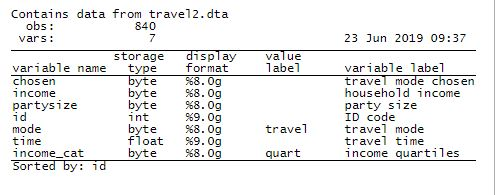To begin our analysis of these choice data, we tell Stata that the id variable identifies the cases (individuals) and the mode variable identifies the alternatives (modes of travel).

```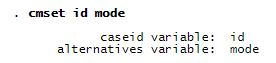```

Before fitting a model, let’s learn a little more about our data. cmtab shows us the proportion of individuals that chose each model of transportation. The option choice(chosen) says that the variable named chosen in our dataset records each individual’s chosen travel mode.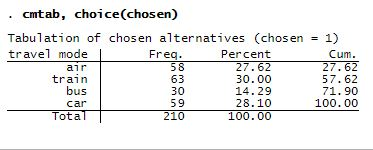Train transportation was most frequently chosen, but just slightly more often than air or car transportation. Only 14% of individuals took a bus.

We are interested in the effect of income on the chosen travel mode. Before we fit our model, let’s look at the mean income for those who selected each travel mode.

```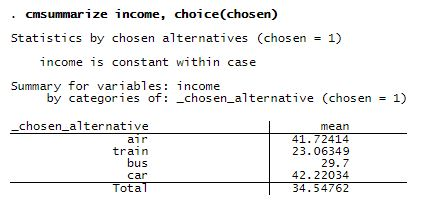```

Not surprisingly, average income is the highest among those who choose to travel by air and by car.

Now, let’s fit a model and see what the results tell us about this relationship and others. To demonstrate, we fit a conditional logistic regression model using cmclogit, but the analysis that follows could be performed after fitting any other choice model.

We will include travel time, income, and the number of individuals traveling together (partysize) as covariates in our model. Travel time differs for each mode of transportation; it is called an alternative-specific variable. Income and party size do not vary across alternatives for an individual; they are known as case-specific variables and are listed in the casevars() option.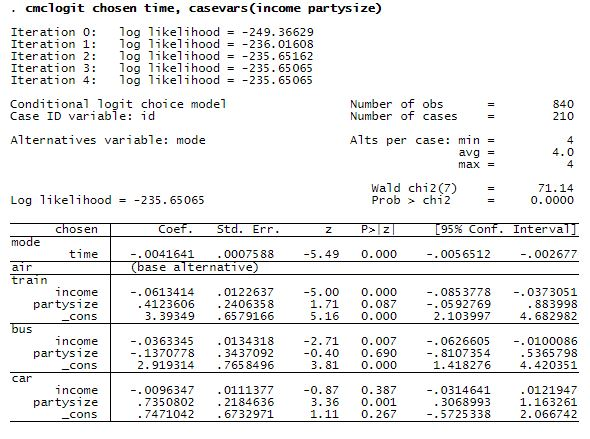What can we determine from these results? The coefficient on time is negative, so the probability of choosing any method of travel decreases as its travel time increases.

For the train alternative, the coefficient on income is negative. Because air travel is the base alternative, this negative coefficient tells us that as income increases, people are less likely to choose a train over an airplane. For the car alternative, the coefficient on partysize is positive. As party size increases, people are more likely to choose a car over an airplane.

But what about questions that we can’t answer from this output? For instance, what happens to the probability of selecting car travel as income increases? This is where margins comes in.

Let’s estimate the expected proportion of travelers who would choose car travel across income levels ranging from \$30,000 to \$70,000 per year.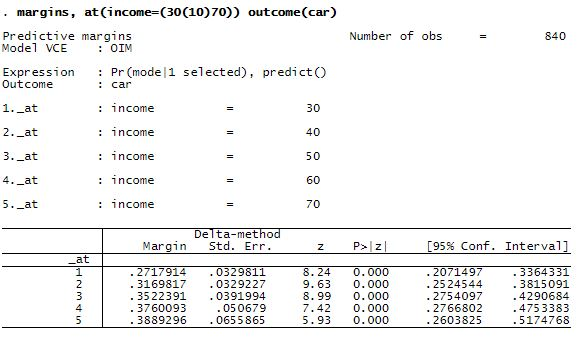In the first line, we see that at the \$30,000 income level, 27% of travelers are expected to choose car travel. But at the \$70,000 income level, 39% are expected to choose car travel.

If we simply type marginsplot, we can easily visualize the effect of income.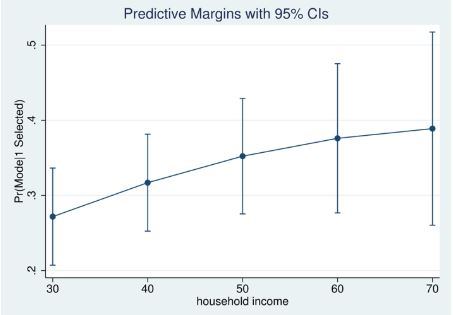We see that the expected probability of choosing car transportation increases as income increases. But are these differences statistically significant?

We can again use margins to answer this question. Specifying the contrast() option, we can test for differences in the expected probabilities for each \$10,000 increase in income. The atcontrast(ar) option requests reverse adjacent contrasts—comparisons with the previous income level.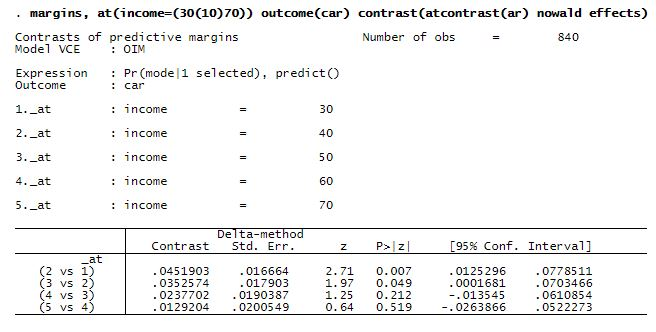At a 5% significance level, the effect of a \$10,000 increase in income is statistically significant only when going from \$30,000 to \$40,000 (2 vs 1) and from \$40,000 to \$50,000 (3 vs 2).

Above, we have results for car travel, but we can easily estimate similar effects for each other travel alternative. We simply change outcome(car) to outcome(train) or any of the other outcomes. Or we can remove the outcome() option altogether and get estimates for all outcomes. This is helpful if you want to compare effects of income across all modes of transportation. For instance, if we type

. margins, at(income=(30(10)70)) . marginsplot, noci

we see how the expected probability of selecting each transportation mode changes across income levels.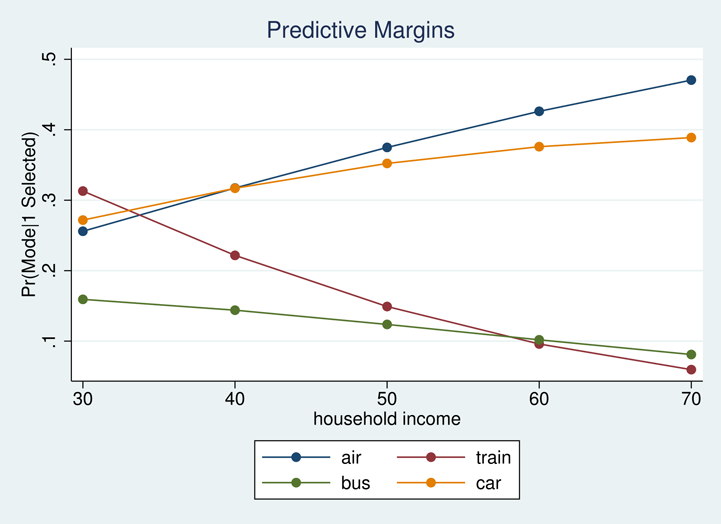So far, we have focused on the effects of income. What about analyzing the effect of an alternative-specific variable like time? We can ask different questions for these variables. For instance, if wait times at airports increase by an hour, how do we expect this to affect the probability of selecting air travel? How does it affect the probability of selecting car travel? Train travel? Bus travel?

We find out by typing

. margins, at(time=generate(time)) at(time=generate(time+60)) alternative(air)

The first at() option requests expected probabilities without the additional travel time, and the second at() option requests expected probabilities with an additional 60 minutes added to air travel time.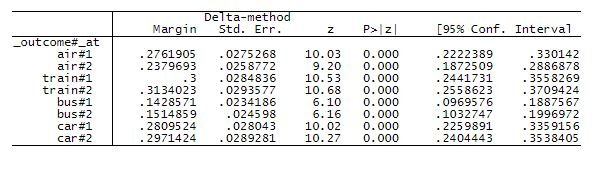Not surprisingly, we see a decrease in the expected probability of traveling by air from 0.276 to 0.238. The expected probabilities of selecting any of the other travel modes increase slightly.

Again, we can use marginsplot to visualize the effect of this change in air travel time.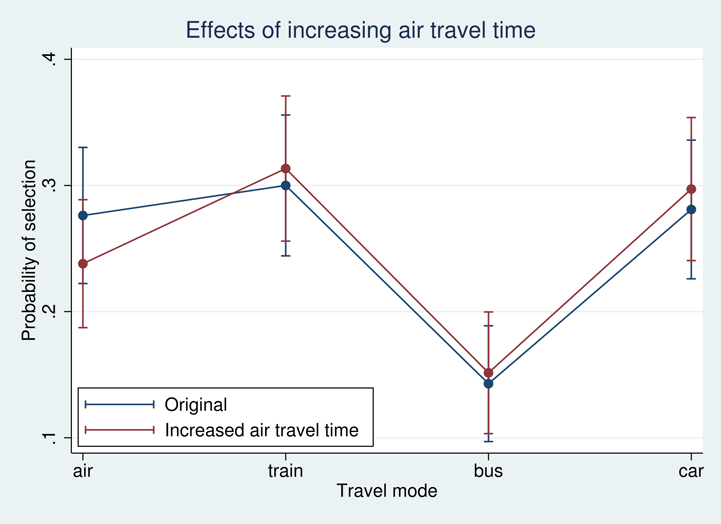Here we have given you only a taste of the types of analyses you can now perform using margins.